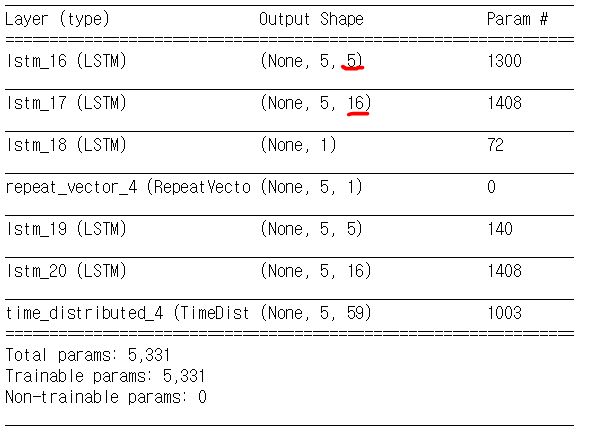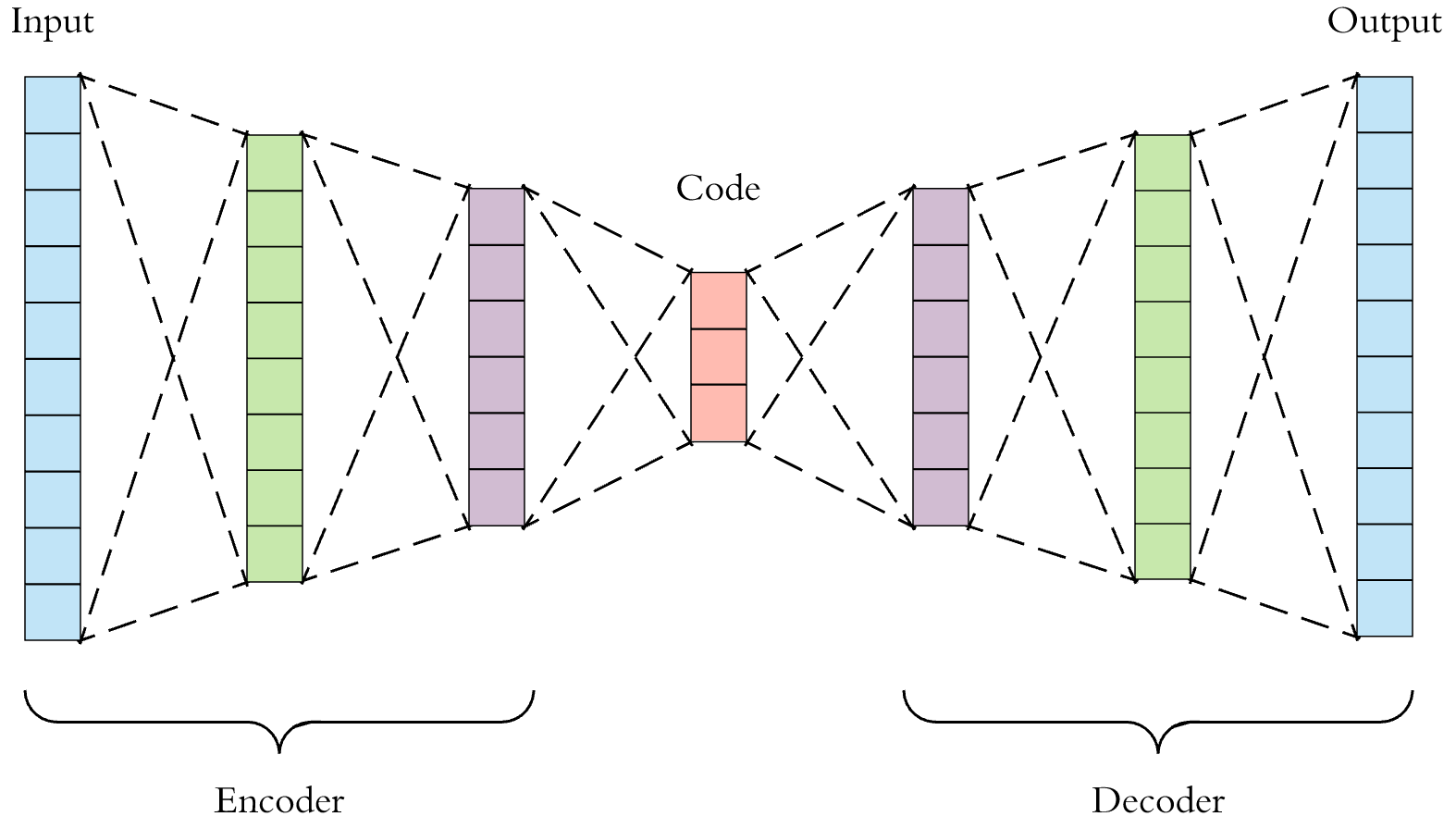# How smaller does the input data get reduced in a LSTM autoencoder

## Question

1. In a LSTM autoencder, how smaller does my input data(59 features) get reduced in a latent vector, which is usually located in the middle between an encoder and a decoder?

2. Why did the author increase the feature number from 5 to 16 in the middle of encoding stage. This question is described in more detail below after the picture of LSTM autoencoder structure.

My questions are based on the article LSTM Autoencoder for Extreme Rare Event Classification in Keras. You can take a look at the codes from this github repository. Please refer to these resources to get to know my questions better.

## Details of the Question

1. My Autoencoder model is as follows:
lstm_autoencoder = Sequential()

# Encoder

# Decoder

lstm_autoencoder.summary()

1. The shape of the input data is X_train_y0_scaled.shape = (11692,5,59). It means that we have 11692 batches. Each batch is comprised of 5 rows and 59 columns, and since the data is time series data, it means that 59 features are gathered for each of 5 days.

2. The summary of the autoencoder model is as follows:- Side question : I cannot understand why the author of this codes increased the feature number from 5 (in the layer 'lstm_16') to 16 (in the layer 'lstm_17').
- The original number of feature is 59, so in the first layer the feature number got reduced from 59 to 5. But after this dimension reduction, why would someone want to increase the dimension in the encoding stage?!

1. It is easy to see how smaller the shape of the input data gets reduced in a latent vector, if it is a fully-connected autoencoder. For example, in the picture below 10-node long input data gets reduced to 3-node long latent vector.2. However in the LSTM autoencoder, it is not clear to me how long the 59 feature-long vector got reduced to. The layer lstm_18 is only 1-node long while the repeat vector is (5,1) long. Does that mean that 59-feature long vector got reduced to 1-node long vector?